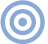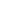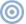# Features

## Measuring Loudspeakers, Part Two The Fast Fourier Transform

Sidebar: The Fast Fourier Transform
As I have implied in this article, a representation of a signal in the time domain has a related representation in the frequency domain. There is a mathematical operation, the Fourier Transform, that can be used to calculate the frequency-domain representation—the signal's spectrum—from its time-domain representation—the signal's impulse response. In practice, a mathematical shortcut is used, leading to the designation of the mathematical operation as the Fast Fourier Transform or FFT (footnote 1).

The basic premise behind the application of the FFT to a loudspeaker's time-domain behavior is that impulse response be first "windowed" to reject data that are not of interest (room reflections, for example). It is then assumed that the impulse response is part of a continuous repetitive waveform, the end of the data window being spliced to its beginning to produce an infinite series of copies of the impulse response. Applying the FFT produces a line spectrum with its first component having a frequency equal to the reciprocal of the time window.: ie, if the impulse response is windowed to have a length of 4ms, the fundamental frequency of the transformed line spectrum is (1/0.004)Hz = 250Hz. The spectral lines present at integer multiples of that 250Hz fundamental—500Hz, 750Hz, 1000kHz, 1250Hz, 1500Hz, etc.—paint out the frequency spectrum of the signal.

It is important to note that no information is present in that spectrum below the fundamental frequency. Nor is any information present between the spectral lines. The windowing operation necessary to perform the FFT eliminates real information. And the shorter the time window, the higher the fundamental frequency in the resultant spectrum and the larger the gaps in that spectrum.—John Atkinson

Footnote 1: In the PC dark ages of the early '80s, I wrote a mixed Basic/assembler FFT program that took two minutes to calculate a 1024-point FFT on a 6502-based computer. But in these days of 300MHz Pentiums and G3 Macs, the FFT operation is truly fast, that same 1024-point FFT taking milliseconds, if not microseconds. Even 32,768-point FFTs, which would have taken hours in 1982, are now commonplace. (For the jitter spectral analyses that accompany Stereophile's CD player reviews, for example, I average 64 32,768-point FFT calculations in a matter of seconds.)—John AtkinsonARTICLE CONTENTSX